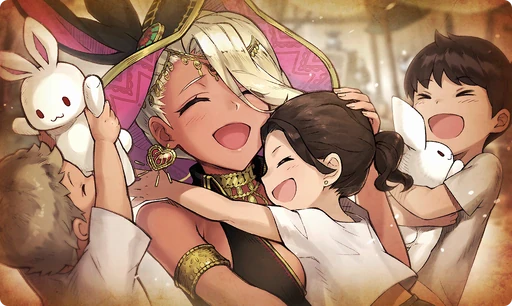# A Special DayOur big sis Strie came home from working out of town. I was so happy to see her. "Hehe, I brought presents!" It was a bunny plushie that had ears like the ones on her hat. We all want to buy her a present one day, because she always gets us stuff. (Samine, To Someone Special, Aira Orphanage Creative Writing Assignment)

### A Special Day

Raises HP by 30%/Strong vs Humans by 20%/Strong vs Gluttony 20% for the Memento group "Desert Dwellers"

Target Buff (SelfSide) (CardLsSkill)
Stats
TypeMinMax
HP+30%+30%
Strong vs Human+20+20
Strong vs <暴食>+20+20

## Stats

#### Simple stats

TypeLvl 1Lvl 20Lvl 30
PATK+7+15+20

LevelPATK
1+7
2+7
3+7
4+8
5+8
6+9
7+9
8+10
9+10
10+11
LevelPATK
11+11
12+11
13+12
14+12
15+13
16+13
17+14
18+14
19+15
20+15
LevelPATK
21+15
22+16
23+16
24+17
25+17
26+18
27+18
28+19
29+19
30+20

## Group skills

### A Hidden Desire

#### Simple stats

TypeLvl 1Lvl 20Lvl 30
PDEF+10+23+30
MDEF+10+23+30

LevelPDEFMDEF
1+10+10
2+10+10
3+11+11
4+12+12
5+12+12
6+13+13
7+14+14
8+14+14
9+15+15
10+16+16
LevelPDEFMDEF
11+16+16
12+17+17
13+18+18
14+18+18
15+19+19
16+20+20
17+21+21
18+21+21
19+22+22
20+23+23
LevelPDEFMDEF
21+23+23
22+24+24
23+25+25
24+25+25
25+26+26
26+27+27
27+27+27
28+28+28
29+29+29
30+30+30

#### Limit Break

TypeLB1LB2LB3LB4LB5
PDEF+4+7+11+14+18
MDEF+4+7+11+14+18

TypeLB5
HP+200